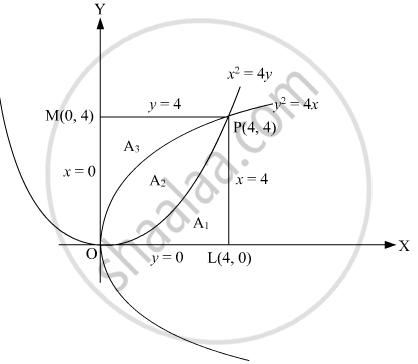# Prove that the curves y^2 = 4x and x^2 = 4y divide the area of square bounded by x = 0, x = 4, y = 4 and y = 0 into three equal parts. - Mathematics

Sum

Prove that the curves y2 = 4x and x2 = 4y divide the area of square bounded by x = 0, x = 4, y = 4 and y = 0 into three equal parts.

#### SolutionA(OABC) = 4 × 4 = 16 sq. units

From, y2 = 4x and x2 = 4y

(x^2/4)^2 = 4x

or     x^4/16 = 4x

or x4 - 64x = 0

or x(x3 - 64) = 0

or x = 0 or x = 4

when x = 0, y = 0

x = 4, y = 4

Point of intersection of the two parabolas is (0, 0) and (4, 4).

Area of part III = int_0^4 y dx (parabola x2 = 4y)

= int_0^4 (x^2)/4 dx = [1/4 x^3/3]_0^4

= 1/12(64 - 0)

= 64/12

= 16/3 sq.units

Area of I = Area of square - Area of II and III

= 16 - int_0^4 sqrt(4x) dx

= 16 - (2 × 2)/3 [x^(3/2)]_0^4

= 16 - 32/3 sq. units

= 16/3 sq. units

Area of II = Area of square - Area of I - Area of III

= 16 - 16/3 - 16/3 sq. units

= 16/3 sq. units

The two curves divide the square into three equal parts.

Concept: Area of the Region Bounded by a Curve and a Line
Is there an error in this question or solution?